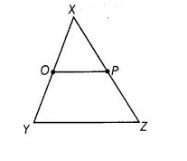`
Question:

In the adjoining figure, if OX = ½ XY, PX = ½ XZ and OX = PX, show that XY = XZ.Solution:

Given OX= ½ XY => 2 OX = XY ……….(i)

PX= ½ XZ => 2 PX = XZ …….(ii)

and OX = PX …(iii)

According to Euclid’s axiom, things which are double of the same things are equal to one another.

On multiplying Eq. (iii) by 2, we get

2 OX = 2 PX

XY=XZ. [from Eqs. (i) and (ii)]